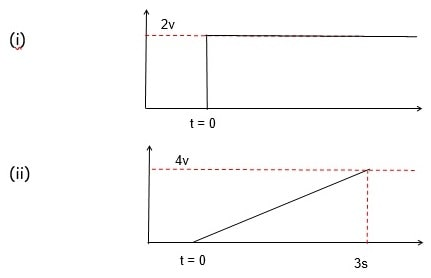# Control System Dynamics assignment question

## B. Eng. Electrical Engineering HNC/D in Engineering

Learning Outcomes

On successful completion students will be able to:

1. be familiar with mathematical methods of modelling control systems
2. understand problems of stability in closed loop control steps in design of compensation to meet a required specification.
3. understand the methods of modern control theory.

Scenario:

The accurate control of real systems is critical for many reasons. These include:

Energy Efficiency and minimal material wastage

Minimal wear on process elements and control elements

Safety.

It is the role of the Control Engineer to ensure that processes are maintained at their optimum conditions and when disturbances occur their effect is minimised.

The Engineer must have a grasp of the theory behind “good” control as well as the supporting mathematics and enabling software.

Specification:

You are required in this assignment to investigate the operation of given systems and to analyse their operation. This will involve mathematical calculation and the construction of graphical representations of systems.

1. a) A system consists of a gain of 5 and two first order lags. The time constants of the lags are 0.125 s and 0.5 s respectively.

Calculate and plot the response of the system to the following inputs:1. b) A controller of gain Kc is added and the loop is closed.

Determine the value of Kc for a damping factor (ζ) of:

• Unity (1) critical
• One over root 2 (1/√2) optimum
• Half (0.5) under damped.

For each of the damping factors determine the steady state error and suggest a generalised statement relating Kc, ζ and the steady state error.

1. c) Over the range 0 to 1 vary Kc and show how the roots vary in the s plane.
1. d) A second order system has a transfer function of .

Determine its response to a step input of 5 units and plot your results. A first order system is added into the process, its transfer function is given by . Determine mathematically the effect on the system if ‘a’ has the following values:

• 20
• 8

In each case plot the new response - what can be said about the added first order pole?

An open look system has the following angular frequencies applied and the respective gains and phase shifts are measured.

 ω Gain dB Phase 1 11.87 -103.2 2 5.36 -115.6 4 -2.22 -136.3 7 -9.80 -157.6 10 -15.41 -171.9 20 -27.88 -199.7 40 -42.57 -226.0 70 -55.90 -242.7 100 -64.82 -250.4 200 -82.60 -260.0 400 -100.59 -265.0 700 -115.15 -267.1 1000 -124.44 -268.0
1. a) Determine an approximate transfer function for the system by plotting the Bode plot and making measurements from the response.
1. b) Apply the four rules of thumb and determine what the response of the system would be if the loop was closed.
1. c) Determine the damping factor and the bandwidth. Would there be any offset (explain why there is or is not).

A Type 2 system has a Transfer Function of the following form:

This system is unstable and therefore uncontrollable. The desired characteristics for the system are:

Percent overshoot ≈ 10%

Rapid roll off at high frequencies.

Design a lead lag compensator to achieve this.